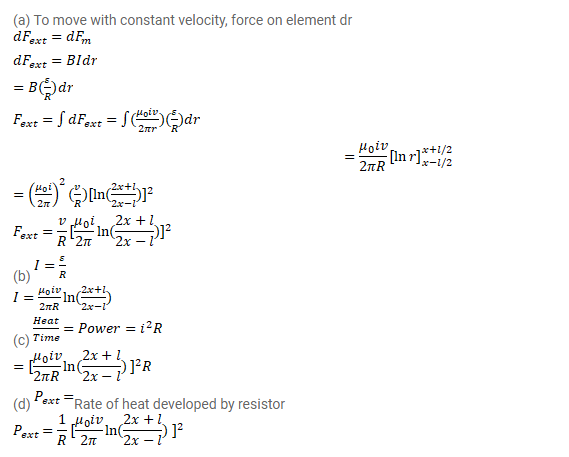# Consider a situation similar to that of the previous problem except that

Question:

Consider a situation similar to that of the previous problem except that the ends of the rod slide on a pair of thick metallic rails laid parallel to the wire. At one end the rails are connected by resistor of resistance R.

(a) What force is needed to keep the rod sliding at a constant speed $v$ ?

(b) In this situation what is the current in the resistance R?

(c) Find the rate of heat developed in the resistor.

(d) Find the power delivered by the external agent exerting the force on the rod.

Solution: